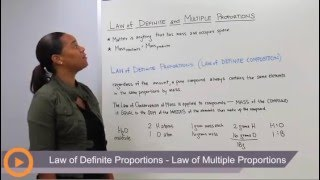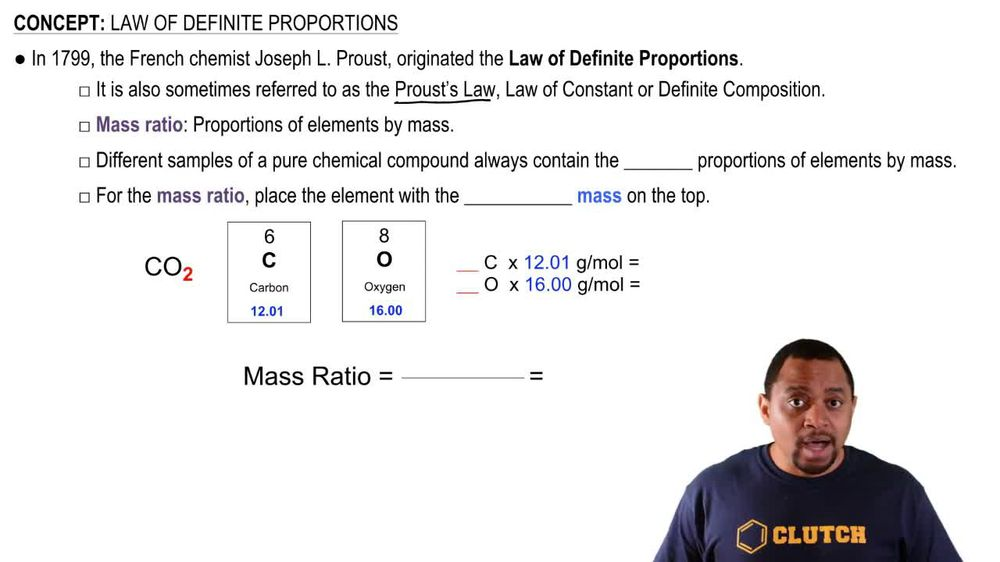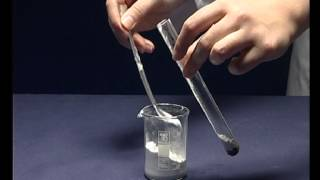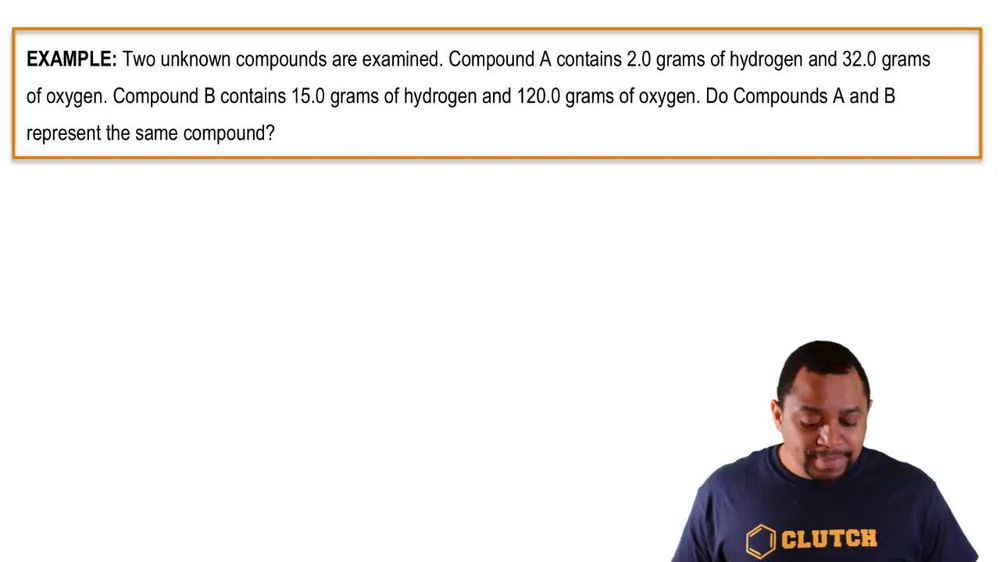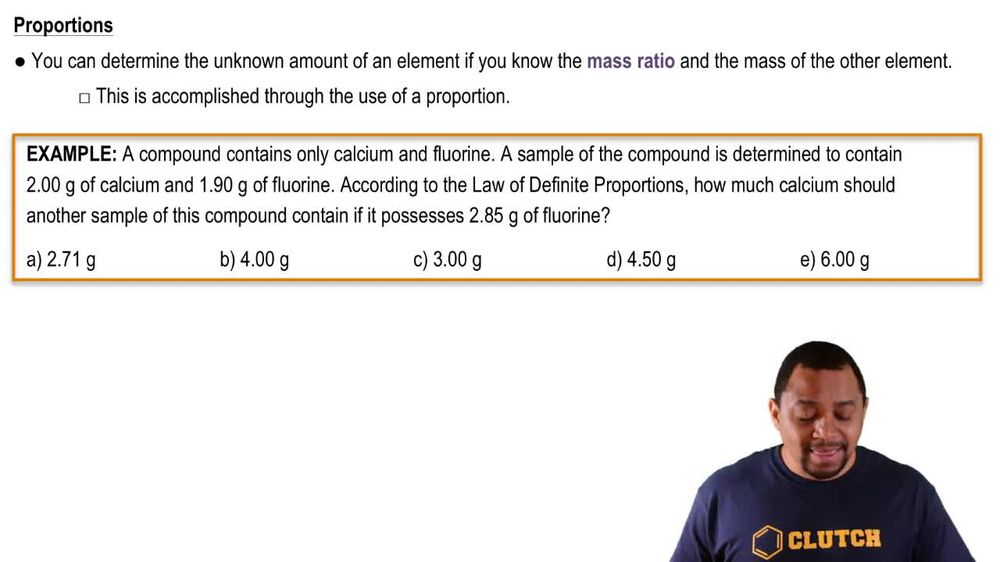Start typing, then use the up and down arrows to select an option from the list.
1. 2. Atoms & Elements2. Law of Definite Proportions
Problem

# A 7.83 g sample of HCN contains 0.290 g of H and 4.06 g of N. Find the mass of carbon in a sample of HCN with a mass of 3.37 g.

Relevant Solution4m
Play a video:
welcome back everyone in this example. We have a 9.78 g sample of ibuprofen. And over the counter pain medication which contains 7.4 g of carbon and 1.52 g of oxygen. We need to find the mass of hydrogen in a 5.4 or 5. g sample of ibuprofen. So this is going to be a mass ratio type of problem. Where because the prompt focuses on our hydrogen atom, we want to recall that the proportion of hydrogen to the sample mass should be the same for every sample of our ibuprofen. So right now we want to figure out our total mass based on our given masses of oxygen, carbon and hydrogen. So we have for our total mass we have carbon plus hydrogen plus oxygen. So from the prompt we have 7.40 g of carbon plus we don't know how many grams of carbon or hydrogen we have. So we're going to say x grams of hydrogen And then plus our massive oxygen we're given is 1.52 g of oxygen. And so we are actually given the total mass of ibuprofen from the prompt as that value, 9.78 g of ibuprofen. We'll use I bu as our shorthand for ibuprofen. And we don't know our massive hydrogen. So we need to solve for our massive hydrogen. So we would just take the difference here and say that our mass of hydrogen is going to equal our 9.78 g of ibuprofen which we subtract from our massive carbon. So 7.40 g of carbon and subtracting that from our 1.52 g of oxygen. And so this is going to give us a difference for our massive hydrogen equal to a value of 0.860 g as our mass of hydrogen. And so we want to find the proportion of this massive hydrogen to each of our samples of ibuprofen and see if those values are consistent. And so making note of our mass ratio here. Or we can just say our proportion of hydrogen to our ibuprofen. We have our hydrogen mass being 8.60 g divided by our mass of ibuprofen given from the prompt as 9.78 g. And this gives us a proportion equal to a value of 0.879. So we're going to compare our second calculation to see if we get the same proportion to our law of definite proportions. And so we would say that we have our mass of hydrogen In a 5.45 g sample, Which we can calculate by taking our above value as 0.860 g. We're going to divide it by the mass of the original ibuprofen sample being 9. g of ibuprofen. So this is our mass of hydrogen divided by our massive ibuprofen. And we are going to multiply this quotient by our mass of our second sample of ibuprofen given as 5. g of ibuprofen. And so this allows us to get our mass of hydrogen for our second sample equal to a value of 0.479 g of our hydrogen. And so we want to see if we get the same proportion as above to prove our law of multiple proportions. So we're going to take our mass of hydrogen again divided by our mass of ibuprofen according to the second sample. And above that would be 0.479 g of hydrogen divided by our massive ibuprofen. For the second sample being 5.45 g and sorry 5.45 g of ibuprofen. And this gives us a proportion equal to a value of also 0.879. And so this proves that our law proportion applied here because we have the same proportion of our massive hydrogen to mass of ibuprofen. And so for our final answer, we can confirm that our massive hydrogen is equal 2.479 g in the 5.45 g sample of ibuprofen. So I hope that everything I've read was clear. If you have any questions, please leave them down below And I will see everyone in the next practice video